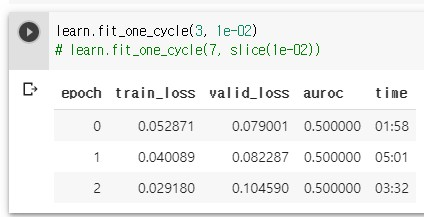# AUROC, Dice and Accuracy in metrics do NOT work

Hi all,

I’m trying to use Fast.AI Tabular with Dice and Accuracy as metrics, but they do not work.

1. AUROC
It works, but the result is weird.
Every epoch shows 0.5 AUROC, even when I tried 20 epochs.
But, when I calculated AUROC with valid values manually(with sklearn package), it was not 0.5, but about 0.8.How can I fix this?

1. Dice
When I run, I get this error.

However, my data set has only float and category. I don’t know what “Long” is in my data.
Of course, I tried to change “category” to “float” because those columns have numbers as a category, but the result is the same.

2. Accuracy
When I run with “accuracy” as a metric, I get this error.

As you can see, it requires “Long” but it says that my data has “float”
It’s totally opposite to what I got in “dice”. It’s really weird.

Among the three metrics, AUROC is the most important to me now because I have to submit my result with this metric.

And this is my code for fastai and dataset.

dep_var = ‘isFraud’
cat_names = [e for e in cat if e in train.columns.tolist()]
cont = train.columns.tolist()
cont_names = [e for e in cont if e not in (cat)]
cont_names.remove(‘isFraud’)
procs = [FillMissing, Categorify, Normalize]
path = base_dir

data = TabularDataBunch.from_df(path, train, dep_var,procs =procs, valid_idx=val_idx, test_df=test, cat_names=cat_names, cont_names=cont_names)

learn = tabular_learner(data, layers=[50,25], metrics=[AUROC()], y_range=[0,1])
#or
learn = tabular_learner(data, layers=[50,25], metrics=[accuracy], y_range=[0,1])

learn.fit_one_cycle(7, 1e-005)